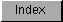```
The  sampling period  is limited by the number of DMA cycles allocated to
an audio channel. Each audio channel is allocated one DMA slot per
horizontal scan line of the screen display. An audio channel can retrieve
two data samples during each horizontal scan line. The following
calculation gives the maximum sampling rate in samples per second.

2 samples/line * 262.5 lines/frame * 59.94 frames/second = 31,469
samples/second

The figure of 31,469 is a theoretical maximum. In order to save buffers,
the hardware is designed to handle 28,867 samples/second. The system
timing interval is 279.365 nanoseconds, or .279365 microseconds. The
maximum sampling rate of 28,867 samples per second is 34.642 microseconds
per sample (1/28,867 = .000034642). The formula for calculating the
sampling period  is:

sample interval     clock constant
Period value = --------------- = ------------------
clock interval    samples per second

Thus, the minimum period value is derived by dividing 34.642 microseconds
per sample by the number of microseconds per interval:

34.642 microseconds/sample
Minumum period = ------------------------------ = 124 timing
0.279365 microseconds/interval   intervals/sample

or:

3,579,545 ticks/second
Minumum period = ----------------------  = 124 ticks/sample
28,867 samples/second

Therefore, a value of at least 124 must be written into the
period register  to assure that the audio system DMA will be able to
retrieve the next data sample. If the period value is below 124, by the
time the cycle count has reached 0, the audio DMA will not have had
enough time to retrieve the next data sample and the previous sample will
be reused.

28,867 samples/second is also the maximum sampling rate for PAL systems.
Thus, for PAL systems, a value of at least 123 ticks/sample must be
written into the  period register .

Clock Values
------------

NTSC        PAL     units
----        ---     -----
Clock Constant    3579545    3546895   ticks per second
Clock Interval   0.279365   0.281937   microseconds per interval

NOTE:
-----
The Clock Interval is derived from the clock constant, where:

1
clock interval = --------------
clock constant

then scale the result to microseconds. In all of these calculations
"ticks" and "timing intervals" refer to the same thing.

```